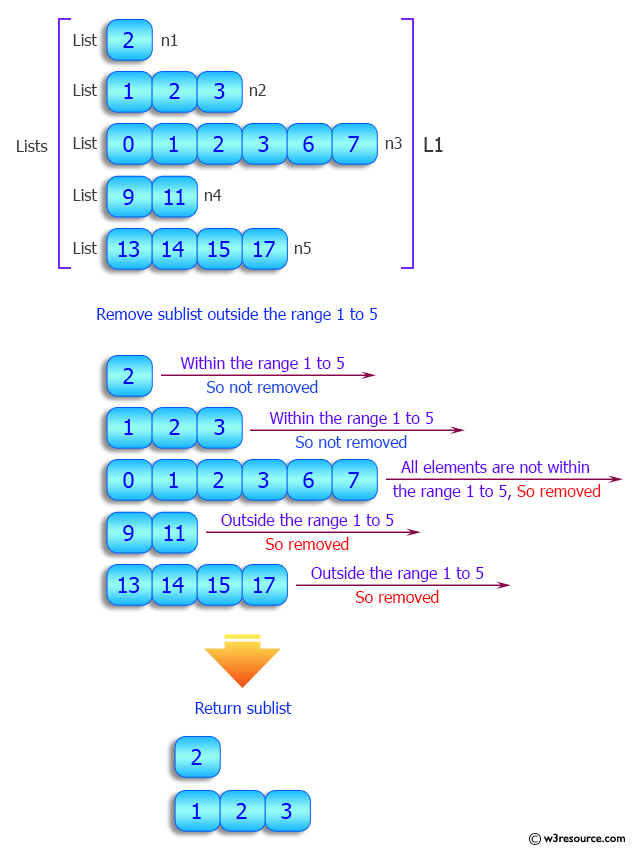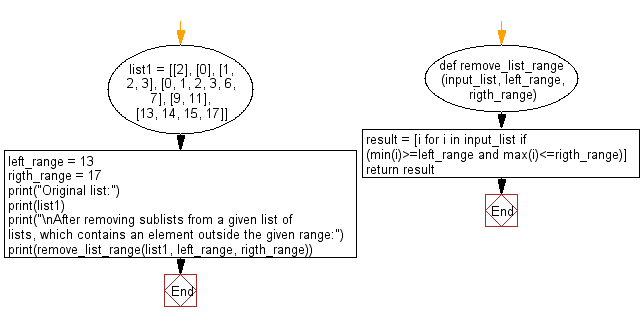﻿ Python: Remove sublists from a given list of lists, which are outside a given range - w3resource# Python: Remove sublists from a given list of lists, which are outside a given range

## Python List: Exercise - 97 with Solution

Write a Python program to remove sublists from a given list of lists, which contains an element outside a given range.

Sample Solution:

Python Code:

``````#Source bit.ly/33MAeHe
def remove_list_range(input_list, left_range, rigth_range):
result = [i for i in input_list if (min(i)>=left_range and max(i)<=rigth_range)]
return result
list1 = [, , [1, 2, 3], [0, 1, 2, 3, 6, 7], [9, 11], [13, 14, 15, 17]]
left_range = 13
rigth_range = 17
print("Original list:")
print(list1)
print("\nAfter removing sublists from a given list of lists, which contains an element outside the given range:")
print(remove_list_range(list1, left_range, rigth_range))
```
```

Sample Output:

```Original list:
[, , [1, 2, 3], [0, 1, 2, 3, 6, 7], [9, 11], [13, 14, 15, 17]]

After removing sublists from a given list of lists, which contains an element outside the given range:
[[13, 14, 15, 17]]
```

Pictorial Presentation:Flowchart:## Visualize Python code execution:

The following tool visualize what the computer is doing step-by-step as it executes the said program:

Python Code Editor:

Have another way to solve this solution? Contribute your code (and comments) through Disqus.

What is the difficulty level of this exercise?

Test your Python skills with w3resource's quiz

﻿

## Python: Tips of the Day

```print(2_000_000)
```2000000